# Reasoning - Seating Arrangement

We have to draw a rough diagram to understand the sitting arrangement − Let’s suppose

B is sitting to the right of A, then we will draw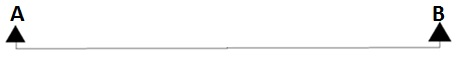If it is given

C is sitting second to the right of A, then we will draw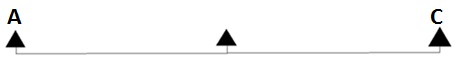If it is given

D is sitting third to the right of A, then we will draw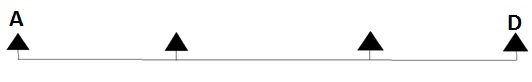• Circular Seating Arrangement
• Linear Seating Arrangement
• Hexagonal Seating Arrangement
• Seating Linear but Facing to each other Arrangement etc.

### Circular seating arrangement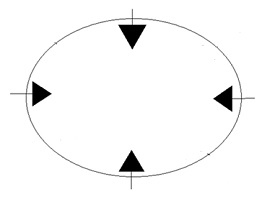In circular sitting arrangement, some conditions are given. We have to follow those conditions and place the required person accordingly in a circular manner. In circular sitting arrangement, we can get question based on

(a) Persons are facing inside the circle.

(b) Persons are facing outside the circle.

(c) Some persons are facing inside and some are facing outside the circle.

For Example

Question Study the given information carefully and answer the following questions.

Six army majors are sitting around a circular table and discussing about stopping infiltration across the border. Major Bakshi is sitting between Major Kalia and Major Sodhi. Major Kalia is sitting immediate left of Major Bakshi. Major Kumar is sitting second to the left of Major Kalia. Major Nanda is sitting between Major Kumar and Major Sodhi.

1 − What is the position of Major Batra?

A − Major Batra is sitting between Major Kumar and Major Kalia.

B − Major Batra is sitting to the left of Major Kalia.

C − Major Batra is sitting to the immediate right of Major Kumar.

D − All the above are true.

Explanation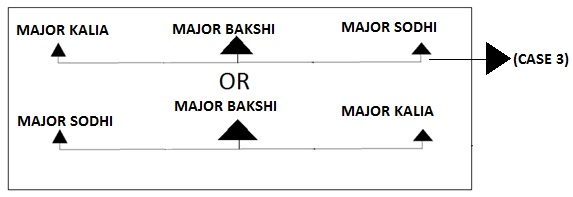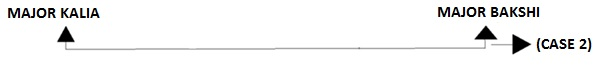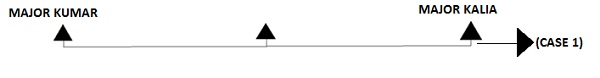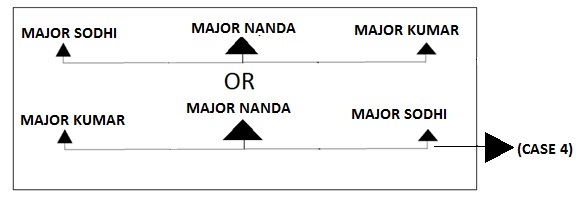By applying (CASE 1), (CASE 2), (CASE 3) and (CASE 4), we get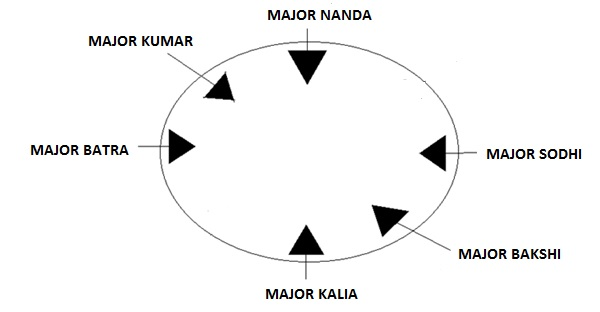All the options (A),(B) and (C) satisfy our condition. Therefore option (D) is correct.

2 − Who is sitting to the immediate left of Major Kumar?

A − Major Bakshi

B − Major Batra

C − Major Nanda

D − Major Sodhi

Explanation − According to the diagram −By observing the diagram, we can clearly say that Major Nanda is sitting to the immediate left of Major Kumar.

3 − Who is sitting to the immediate right of Major Kalia?

A − Major Nanda

B − Major Kumar

C − Major Kalia

D − Major Bakshi

Explanation − According to the diagram −By observing the diagram, one can easily conclude that major Bakshi is sitting to the immediate right of Major Kalia.

4 − Which of the following statement is true?

A − Major Sodhi is sitting second to the left of Major Bakshi.

B − Major Kalia is sitting between Major Nanda and Major Kumar.

C − Major Batra is sitting to the left of Major Kalia.

D − Major Nanda is sitting to the left of Major Kalia.

Explanation − According to the diagram −By observing the diagram, we can conclude that options (A), (B) and (D) do not satisfy the condition. But option (C) does.

5 − How many Majors are sitting between Major Sodhi and Major Kumar, if counted in clockwise direction?

A − Six

B − Two

C − Three

D − Five

Explanation − According to the diagram −Major Bakshi, Major Kalia and Major Batra are the three majors sitting between Major Sodhi and Major Kumar.

6 − What is Major Batra position with respect to Major Sodhi?

A − Second to the left.

B − Immediate Right.

C − Fourth to the Right.

D − Third to the left.

Explanation − According to the diagram −By observing the diagram, we can conclude that Major Bakshi is sitting to the immediate left of Major Sodhi. Major Kalia is sitting to the second left of Major Sodhi and Major Batra is sitting third to the left of Major Sodhi. So, our required answer is option (D).

### Linear Seating Arrangement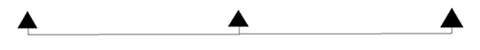In a linear seating arrangement, persons are sitting in a line or row facing north or south direction. Here also some conditions are given on the basis of which we have to get our required answer.

For Example

Question − Study the given information carefully and answer the following questions.

Four friends U, V, W and X are sitting in a row and facing towards north direction. U and X are sitting at two extreme ends. V is sitting between U and W. V is sitting second to the left of X.

1 − Who is sitting to the immediate right of V?

A − X

B − U

C − W

D − None of these

Explanation − According to the diagram −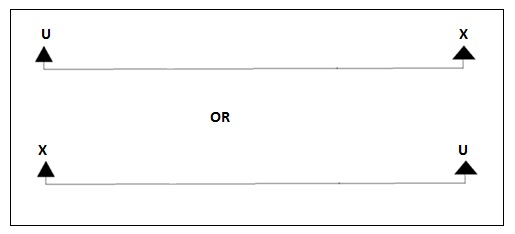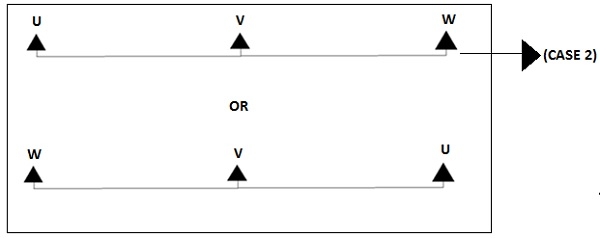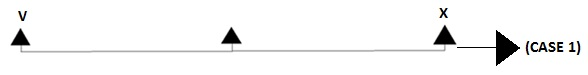By applying (CASE 1) and (CASE 2), we get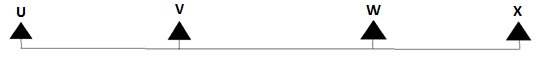From the diagram, it is clear that W is sitting immediate right of V.

2 − Who is sitting to the immediate left of X?

A − W

B − U

C − V

D − None of these

Explanation − According to the diagram −By observing the diagram, we can conclude that W is sitting immediate left of X.

3 − What is the number of People sitting between X and U?

A − Three

B − Five

C − One

D − Two

Explanation − According to the diagram −By observing the diagram, we can conclude that V and W are the two people sitting between U and X.

4 − Which statement is true?

A − X is sitting to the left of U.

B − U and X are sitting at extreme ends.

C − U is sitting between V and X.

D − Three people are sitting between U and X.

Explanation − According to the diagram −U and X are the two people sitting at extreme ends whereas V is sitting to the right of U and W is sitting to the left of X.

5 − Which of the following pairs is the first person sitting to the immediate right of second person?

A − VW

B − WV

C − UV

D − None of these

Explanation − According to the diagram −Here in option (B), the second person is V and the first person is W. So according to the condition, that is the first person sitting to the immediate right of the second person, only satisfies in option (B).

6 − How many persons are there to the right of U?

A − Two

B − Four

C − One

D − Three

Explanation − According to the diagram −By observing the diagram, we can conclude that three person V, W and X are sitting to the right of U.

reasoning_seating_arrangement.htm